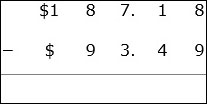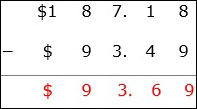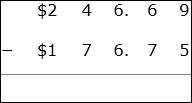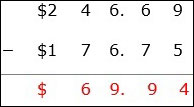# Subtraction With Money

Subtraction of money in dollars and cents is same as the subtraction of decimal numbers.

• The money amounts to be subtracted are lined up vertically with the decimal points aligned one above the other.

• The digits in the rightmost column are first subtracted.

• Then the digits in all columns are subtracted one by one till we get the difference of the money amounts.

• We bring down the decimal point and the dollar sign at the right places.

Subtract $187.18 −$93.49

### Solution

Step 1:The money amounts to be subtracted are lined up vertically with the decimal points and dollar signs aligned one above the other.

Step 2:

We begin at the right most column 8 < 9, so we borrow 1 from left; 1 becomes 0 and 18 − 9 = 9, next 0 > 4, so we borrow from left; 7 becomes 6 and 10 – 4 = 6; next 6 – 3 = 3; and 8 < 9, so we borrow from left, and we have 18 – 9 = 9.

Step 3:

So we get the final difference of the money amounts as $93.69Subtract$246.69 − 176.75 ### Solution Step 1:The money amounts to be subtracted are lined up vertically with the decimal points and dollar signs aligned one above the other. Step 2: We begin at the right most column 9 – 5 = 4; 6 < 7, so we borrow 1 from left; 6 becomes 5 and 16 − 7 = 9, next 5 < 6, so we borrow 1 from left; 4 becomes 3 and 15 – 6 = 9; 3 < 7, so we borrow 1 from left; 2 becomes 1 and we have 13 – 7 = 6; next 1 – 1 = 0 Step 3: So we get the final difference of the money amounts as 69.94